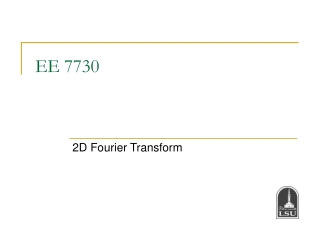DownloadDownload PresentationEE 7730

# EE 7730

Download Presentation## EE 7730

- - - - - - - - - - - - - - - - - - - - - - - - - - - E N D - - - - - - - - - - - - - - - - - - - - - - - - - - -
##### Presentation Transcript

1. EE 7730 2D Fourier Transform

2. Summary of Lecture 2 • We talked about the digital image properties, including spatial resolution and grayscale resolution. • We reviewed linear systems and related concepts, including shift invariance, causality, convolution, etc. EE 7730 - Image Analysis I

3. Fourier Transform • What is ahead? • 1D Fourier Transform of continuous signals • 2D Fourier Transform of continuous signals • 2D Fourier Transform of discrete signals • 2D Discrete Fourier Transform (DFT) EE 7730 - Image Analysis I

4. Fourier Transform: Concept • A signal can be represented as a weighted sum of sinusoids. • Fourier Transform is a change of basis, where the basis functions consist of sines and cosines. EE 7730 - Image Analysis I

5. Fourier Transform • Cosine/sine signals are easy to define and interpret. • However, it turns out that the analysis and manipulation of sinusoidal signals is greatly simplified by dealing with related signals called complex exponential signals. • A complex number: z = x + j*y • A complex exponential signal: r*exp(j*a) =r*cos(a) + j*r*sin(a) EE 7730 - Image Analysis I

6. Fourier Transform: 1D Cont. Signals • Fourier Transform of a 1D continuous signal “Euler’s formula” • Inverse Fourier Transform EE 7730 - Image Analysis I

7. Fourier Transform: 2D Cont. Signals • Fourier Transform of a 2D continuous signal • Inverse Fourier Transform • Fandfare two different representations of the same signal. EE 7730 - Image Analysis I

8. Examples Magnitude: “how much” of each component Phase: “where” the frequency component in the image EE 7730 - Image Analysis I

9. Examples EE 7730 - Image Analysis I

10. Fourier Transform: Properties • Linearity • Shifting • Modulation • Convolution • Multiplication • Separable functions EE 7730 - Image Analysis I

11. Fourier Transform: Properties • Separability 2D Fourier Transform can be implemented as a sequence of 1D Fourier Transform operations. EE 7730 - Image Analysis I

12. Fourier Transform: Properties • Energy conservation EE 7730 - Image Analysis I

13. Fourier Transform: Properties • Remember the impulse function (Dirac delta function) definition • Fourier Transform of the impulse function EE 7730 - Image Analysis I

14. Fourier Transform: Properties • Fourier Transform of 1 Take the inverse Fourier Transform of the impulse function EE 7730 - Image Analysis I

15. Fourier Transform: 2D Discrete Signals • Fourier Transform of a 2D discrete signal is defined as where • Inverse Fourier Transform EE 7730 - Image Analysis I

16. Fourier Transform: Properties • Periodicity: Fourier Transform of a discrete signal is periodic with period 1. 1 1 Arbitrary integers EE 7730 - Image Analysis I

17. Fourier Transform: Properties • Linearity, shifting, modulation, convolution, multiplication, separability, energy conservation properties also exist for the 2D Fourier Transform of discrete signals. EE 7730 - Image Analysis I

18. Fourier Transform: Properties • Linearity • Shifting • Modulation • Convolution • Multiplication • Separable functions • Energy conservation EE 7730 - Image Analysis I

19. Fourier Transform: Properties • Define Kronecker delta function • Fourier Transform of the Kronecker delta function EE 7730 - Image Analysis I

20. Fourier Transform: Properties • Fourier Transform of 1 To prove: Take the inverse Fourier Transform of the Dirac delta function and use the fact that the Fourier Transform has to be periodic with period 1. EE 7730 - Image Analysis I

21. Impulse Train • Define a comb function (impulse train) as follows where M and N are integers EE 7730 - Image Analysis I

22. Impulse Train • Fourier Transform of an impulse train is also an impulse train: EE 7730 - Image Analysis I

23. Impulse Train EE 7730 - Image Analysis I

24. Impulse Train • In the case of continuous signals: EE 7730 - Image Analysis I

25. Impulse Train EE 7730 - Image Analysis I

26. Sampling EE 7730 - Image Analysis I

27. Sampling No aliasing if EE 7730 - Image Analysis I

28. Sampling If there is no aliasing, the original signal can be recovered from its samples by low-pass filtering. EE 7730 - Image Analysis I

29. Sampling Aliased EE 7730 - Image Analysis I

30. Sampling Anti-aliasing filter EE 7730 - Image Analysis I

31. Sampling • Without anti-aliasing filter: • With anti-aliasing filter: EE 7730 - Image Analysis I

32. Anti-Aliasing a=imread(‘barbara.tif’); EE 7730 - Image Analysis I

33. Anti-Aliasing a=imread(‘barbara.tif’); b=imresize(a,0.25); c=imresize(b,4); EE 7730 - Image Analysis I

34. Anti-Aliasing a=imread(‘barbara.tif’); b=imresize(a,0.25); c=imresize(b,4); H=zeros(512,512); H(256-64:256+64, 256-64:256+64)=1; Da=fft2(a); Da=fftshift(Da); Dd=Da.*H; Dd=fftshift(Dd); d=real(ifft2(Dd)); EE 7730 - Image Analysis I

35. Sampling EE 7730 - Image Analysis I

36. Sampling No aliasing if and EE 7730 - Image Analysis I

37. Interpolation Ideal reconstruction filter: EE 7730 - Image Analysis I

38. Ideal Reconstruction Filter EE 7730 - Image Analysis I